# Gallery of Missing Data Visualisations

#### 2023-02-02

There are a variety of different plots to explore missing data available in the naniar package. This vignette simply showcases all of the visualisations. If you would like to know more about the philosophy of the `naniar` package, you should read the vignette Getting Started with naniar.

A key point to remember with the visualisation tools in `naniar` is that there is a way to get the data from the plot out from the visualisation.

# Getting started

One of the first plots that I recommend you start with when you are first exploring your missing data, is the `vis_miss()` plot, which is re-exported from `visdat`.

``````library(naniar)

vis_miss(airquality)``````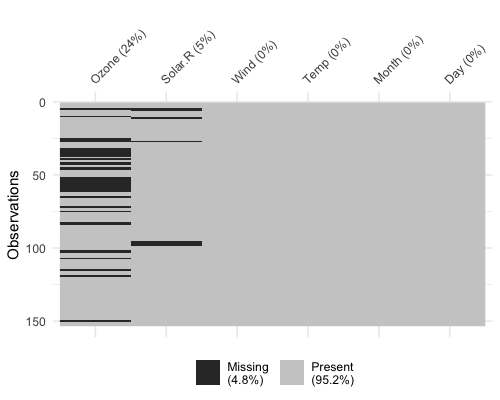This plot provides a specific visualiation of the amount of missing data, showing in black the location of missing values, and also providing information on the overall percentage of missing values overall (in the legend), and in each variable.

## Exploring patterns with UpSetR

An upset plot from the `UpSetR` package can be used to visualise the patterns of missingness, or rather the combinations of missingness across cases. To see combinations of missingness and intersections of missingness amongst variables, use the `gg_miss_upset` function:

``gg_miss_upset(airquality)``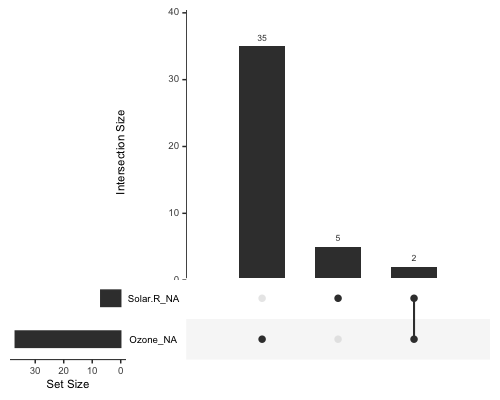This tells us:

• Only Ozone and Solar.R have missing values
• Ozone has the most missing values
• There are 2 cases where both Solar.R and Ozone have missing values together

We can explore this with more complex data, such as riskfactors:

``gg_miss_upset(riskfactors)``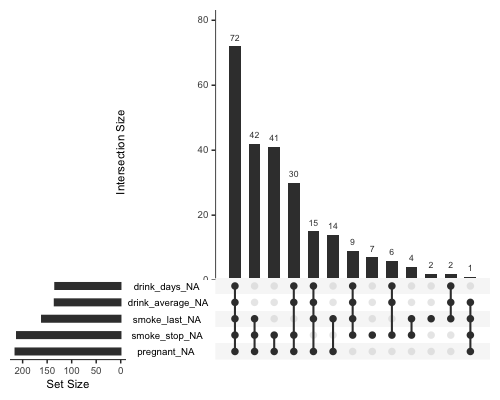The default option of `gg_miss_upset` is taken from `UpSetR::upset` - which is to use up to 5 sets and up to 40 interactions. Here, setting `nsets = 5` means to look at 5 variables and their combinations. The number of combinations or rather `intersections` is controlled by `nintersects`. You could, for example look at all of the number of missing variables using `n_var_miss`:

``````# how many missings?
n_var_miss(riskfactors)``````
``##  24``
``gg_miss_upset(riskfactors, nsets = n_var_miss(riskfactors))``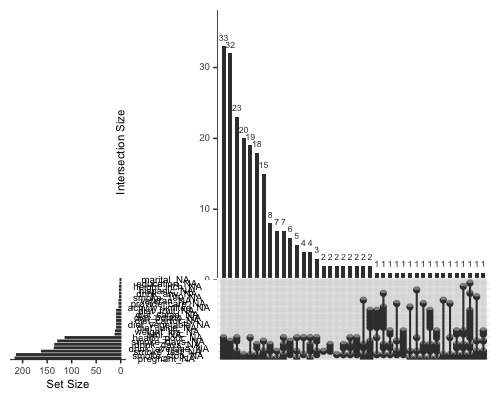If there are 40 intersections, there will be up to 40 combinations of variables explored. The number of sets and intersections can be changed by passing arguments `nsets = 10` to look at 10 sets of variables, and `nintersects = 50` to look at 50 intersections.

``````gg_miss_upset(riskfactors,
nsets = 10,
nintersects = 50)``````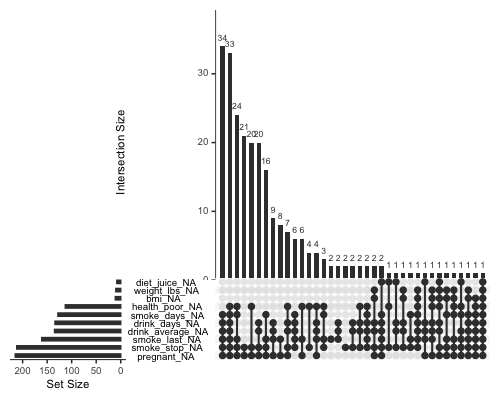Setting `nintersects` to `NA` it will plot all sets and all intersections.

``````gg_miss_upset(riskfactors,
nsets = 10,
nintersects = NA)``````# Exploring Missingness Mechanisms

There are a few different ways to explore different missing data mechanisms and relationships. One way incorporates the method of shifting missing values so that they can be visualised on the same axes as the regular values, and then colours the missing and not missing points. This is implemented with `geom_miss_point()`.

## `geom_miss_point`

``````library(ggplot2)
# using regular geom_point()
ggplot(airquality,
aes(x = Ozone,
y = Solar.R)) +
geom_point()``````
``## Warning: Removed 42 rows containing missing values (`geom_point()`).``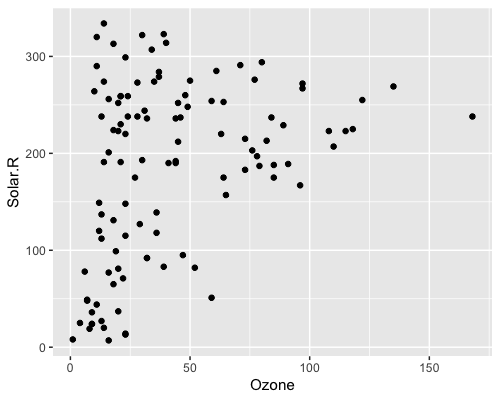``````library(naniar)

# using  geom_miss_point()
ggplot(airquality,
aes(x = Ozone,
y = Solar.R)) +
geom_miss_point()``````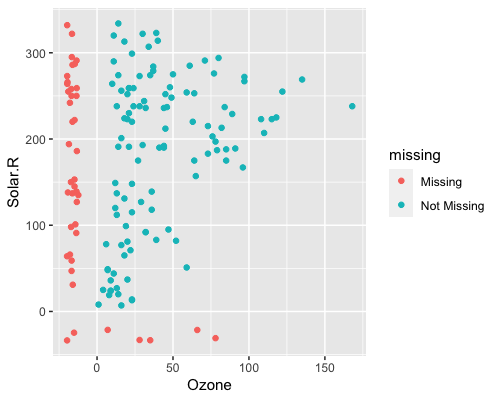``````# Facets!
ggplot(airquality,
aes(x = Ozone,
y = Solar.R)) +
geom_miss_point() +
facet_wrap(~Month)``````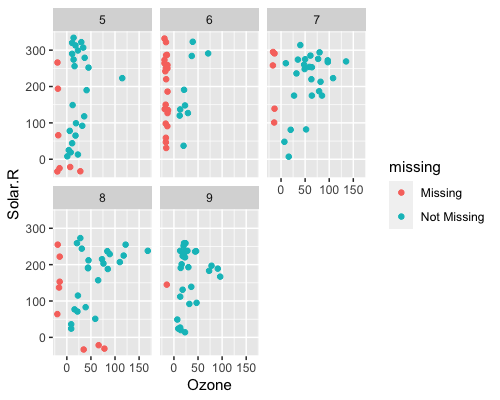``````# Themes
ggplot(airquality,
aes(x = Ozone,
y = Solar.R)) +
geom_miss_point() +
theme_dark()``````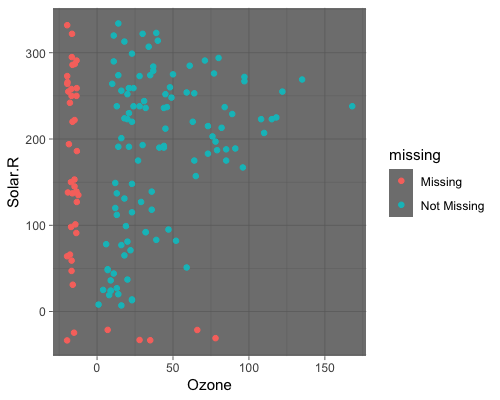# General visual summaries of missing data

Here are some function that provide quick summaries of missingness in your data, they all start with `gg_miss_` - so that they are easy to remember and tab-complete.

## Missingness in variables with `gg_miss_var`

This plot shows the number of missing values in each variable in a dataset. It is powered by the `miss_var_summary()` function.

``gg_miss_var(airquality)``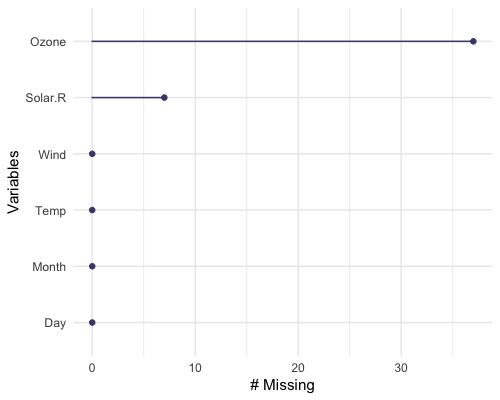``````library(ggplot2)
gg_miss_var(airquality) + labs(y = "Look at all the missing ones")``````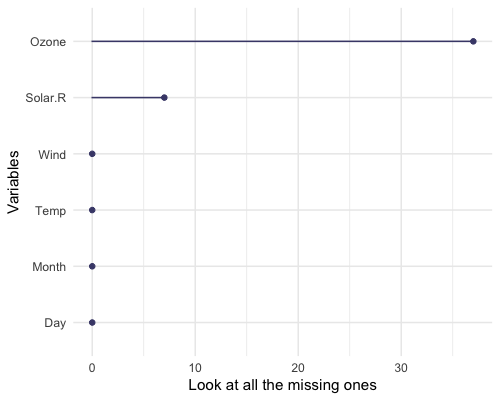If you wish, you can also change whether to show the % of missing instead with `show_pct = TRUE`.

``gg_miss_var(airquality, show_pct = TRUE)``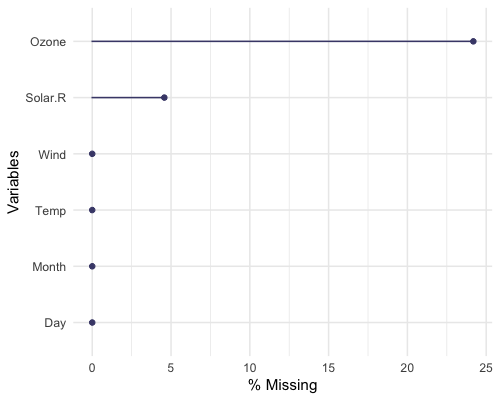You can also plot the number of missings in a variable grouped by another variable using the `facet` argument.

``````gg_miss_var(airquality,
facet = Month)``````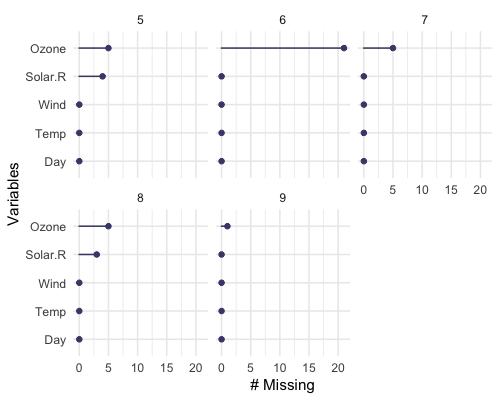## Missingness in cases with `gg_miss_case`

This plot shows the number of missing values in each case. It is powered by the `miss_case_summary()` function.

``gg_miss_case(airquality)``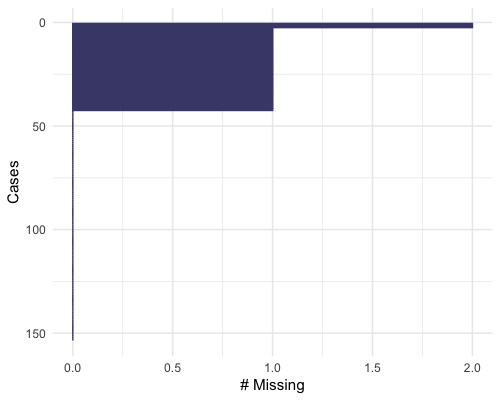``gg_miss_case(airquality) + labs(x = "Number of Cases")``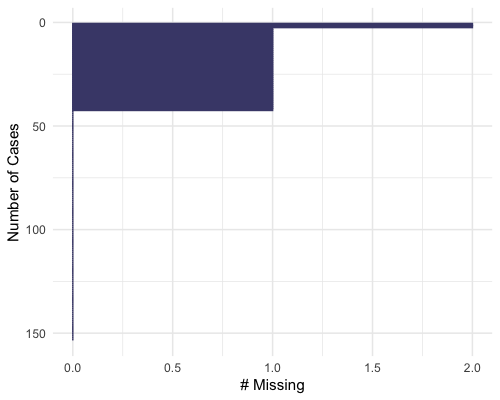You can also order by the number of cases using `order_cases = TRUE`

``gg_miss_case(airquality, order_cases = TRUE)``You can also explore the missingness in cases over some variable using `facet = Month`

``gg_miss_case(airquality, facet = Month)``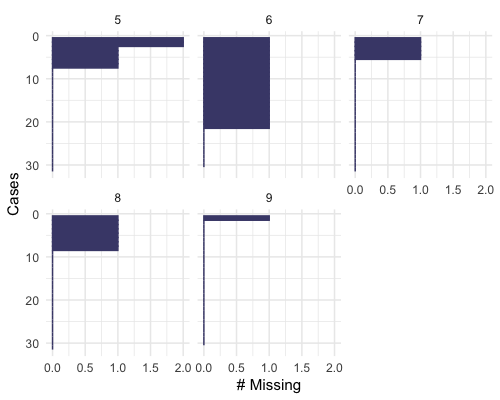## Missingness across factors with `gg_miss_fct`

This plot shows the number of missings in each column, broken down by a categorical variable from the dataset. It is powered by a `dplyr::group_by` statement followed by `miss_var_summary()`.

``gg_miss_fct(x = riskfactors, fct = marital)``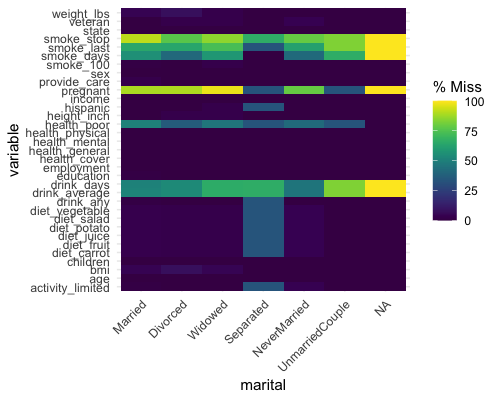``````library(ggplot2)
gg_miss_fct(x = riskfactors, fct = marital) + labs(title = "NA in Risk Factors and Marital status")``````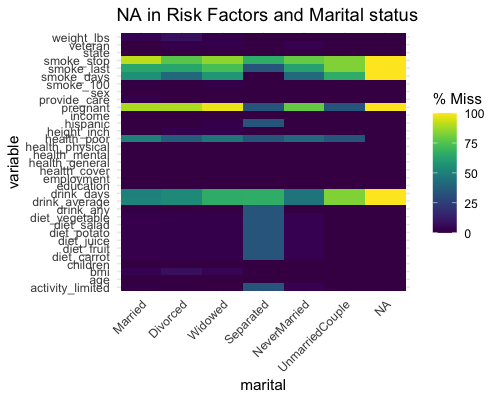``````# using group_by
library(dplyr)
riskfactors %>%
group_by(marital) %>%
miss_var_summary()``````
``````## # A tibble: 231 × 4
## # Groups:   marital 
##    marital variable      n_miss pct_miss
##    <fct>   <chr>          <int>    <dbl>
##  1 Married smoke_stop       120    91.6
##  2 Married pregnant         117    89.3
##  3 Married smoke_last        84    64.1
##  4 Married smoke_days        73    55.7
##  5 Married drink_average     68    51.9
##  6 Married health_poor       67    51.1
##  7 Married drink_days        67    51.1
##  8 Married weight_lbs         6     4.58
##  9 Married bmi                6     4.58
## 10 Married diet_fruit         4     3.05
## # … with 221 more rows``````

`gg_miss_fct` can also be used to explore missingness along time, like so:

``gg_miss_fct(oceanbuoys, year)``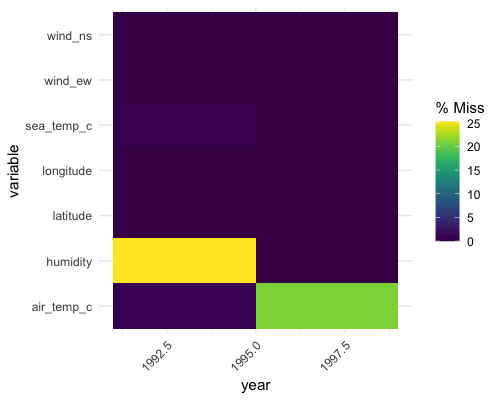``````# to load who data
library(tidyr)
gg_miss_fct(who, year)``````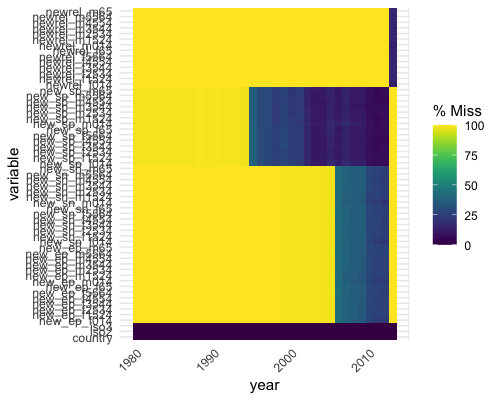(Thanks to Maria Paula Caldas for inspiration for this visualisation, discussed here)

## Missingness along a repeating span with `gg_miss_span`

This plot shows the number of missings in a given span, or breaksize, for a single selected variable. In this case we look at the span of `hourly_counts` from the pedestrian dataset. It is powered by the `miss_var_span` function

``````# data method

miss_var_span(pedestrian, hourly_counts, span_every = 3000)``````
``````## # A tibble: 13 × 6
##    span_counter n_miss n_complete prop_miss prop_complete n_in_span
##           <int>  <int>      <int>     <dbl>         <dbl>     <int>
##  1            1      0       3000  0                1          3000
##  2            2      0       3000  0                1          3000
##  3            3      1       2999  0.000333         1.00       3000
##  4            4    121       2879  0.0403           0.960      3000
##  5            5    503       2497  0.168            0.832      3000
##  6            6    555       2445  0.185            0.815      3000
##  7            7    190       2810  0.0633           0.937      3000
##  8            8      0       3000  0                1          3000
##  9            9      1       2999  0.000333         1.00       3000
## 10           10      0       3000  0                1          3000
## 11           11      0       3000  0                1          3000
## 12           12    745       2255  0.248            0.752      3000
## 13           13    432       1268  0.254            0.746      1700``````
``gg_miss_span(pedestrian, hourly_counts, span_every = 3000)``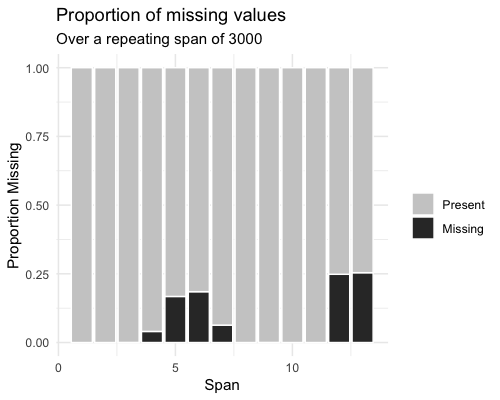``````# works with the rest of ggplot
gg_miss_span(pedestrian, hourly_counts, span_every = 3000) + labs(x = "custom")``````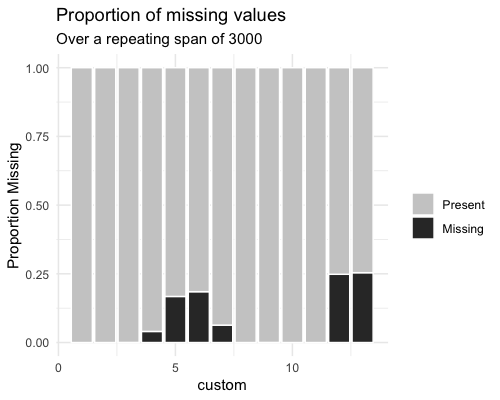``gg_miss_span(pedestrian, hourly_counts, span_every = 3000) + theme_dark()``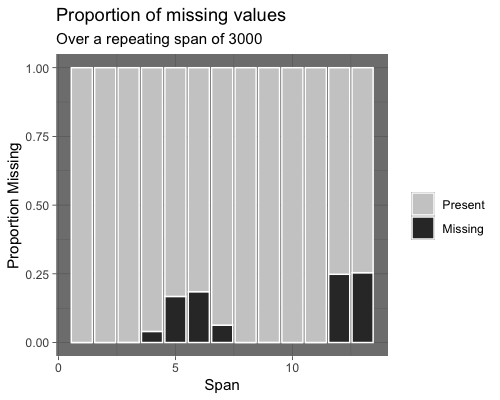You can also explore `miss_var_span` by group with the `facet` argument.

``````gg_miss_span(pedestrian,
hourly_counts,
span_every = 3000,
facet = sensor_name)``````